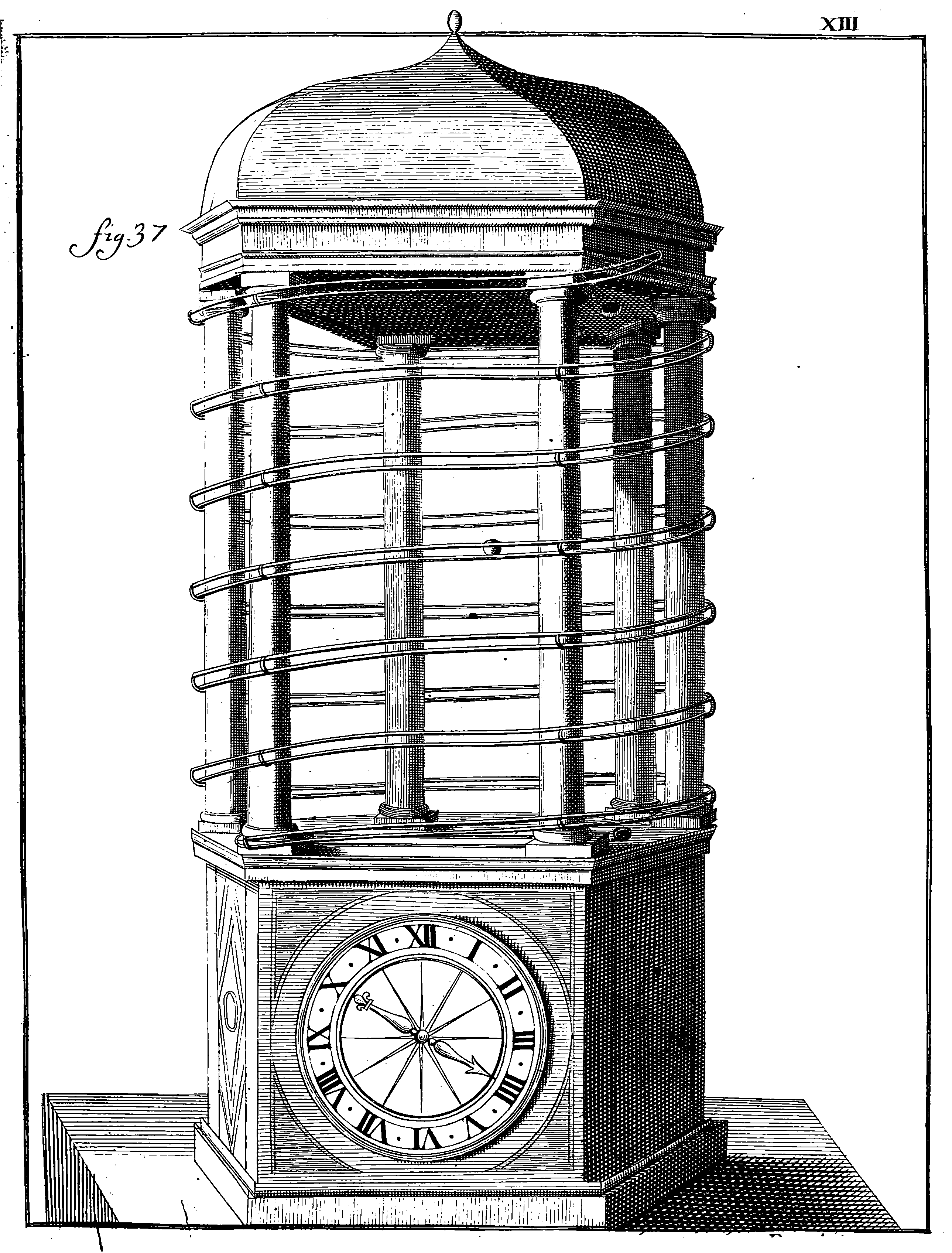# Random change of time

## Stochastic processes derived by varying the rate of time’s passage, which is more convenient than I imagined

🏗 Various notes on a.e. continuous monotonic random changes of index in order build new processes.

In Warping and registration problems you try to align two or more processes; this can sometimes be an alignment problem, but not necessarily.## To explore

Lamperti representation for continuous state branching processes,

Ogata’s time rescaling: Intensity estimation for point processes uses this as a statistical test.

Relation to e.g. martingale transforms.

## Subordinator

A subordinator is a non-decreasing Lévy Process taking values on the reals. AFAICS this is precisely a Gamma process. Curiously, upon giving that definition, many proceed to immediately assert that such a process is a model for a random change of time. This is not insane per se, but doesn’t have much narrative momentum, as a Gamma process can model a bunch of other things than time, and it is a weird specialisation in a field that normally tends to excessive generality.

🏗 I should explain why one would bother doing such an arbitrary thing as changing time though; Basically it is because a time-changed Lévy process is still a Lévy process.

## Point process rate transform

As used in point process residual goodness of fit tests.

A summary in :

Knight showed that for any orthogonal sequence of continuous local martingales, by rescaling time for each via its associated predictable process, we form a multivariate sequence of independent standard Brownian motions. Then Meyer extended Knight’s theorem to the case of point processes, showing that given a simple multivariate point process $${N_i ; i = 1, 2, \dots, n}$$, the multivariate point process obtained by rescaling each $$N_i$$ according to its compensator is a sequence of independent Poisson processes, each having intensity 1. Since then, alternative proofs and variations of this result have been given by Papangelou gave the following interpretation in the univariate case:

Roughly, moving in $$[0, \infty)$$ so as to meet expected future points at a rate of one per time unit (given at each instant complete knowledge of the past), we meet them at the times of a Poisson process. […]

Generalizations of Meyer’s result to point processes on $$\mathbb{R}^d$$ have been established by . In each case, the method used has been to focus on one dimension of the point process, and rescale each point along that dimension according to the conditional intensity.

## Going Multivariate

As seen in, e.g. . How does multivariate time work then?

### No comments yet. Why not leave one?

GitHub-flavored Markdown & a sane subset of HTML is supported.